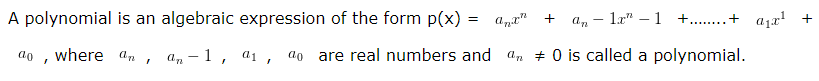# Polynomials Class 10 Maths

Polynomials Class 10 Maths, deals with various concepts which are as under:-

• What are Polynomials?
• Degree of Polynomial
• Types of Polynomials
• Value of a polynomial
• Zero of a polynomial
• Polynomial in one variable – Concept and Examples
• Polynomial coefficients
• Zeroes of Polynomial Examples
• Division of Polynomial with monomial
• Zeroes of Polynomials and Geometry
• Relationship between zeros and coefficient of a polynomial
• Division of a cubic polynomial by monomial
• If a given polynomial is a factor of another polynomial
• Finding all zeroes of biquadratic polynomial
• Finding all zeroes of biquadratic polynomial
• Verification of zeroes of cubic polynomials
• Relation of factors and coefficients of cubic equations
• Finding Zeroes of a Cubic Polynomial

### 1. What are Polynomials?### 2. Degree of Polynomial

The exponent of the highest power of the variable of a polynomial is called the degree of the polynomial.

If”x” is a variable in a polynomial (x), highest power of the variable x in a polynomial is called the degree of the polynomial.

### 3. Types of Polynomials – Polynomials Class 10 Maths

• The polynomial with all the coefficients as zero is called a zero polynomial.
• A polynomial with a single term of a real number is called a constant polynomial.
• A polynomial of degree one is called a first-degree or linear polynomial. the general form of a linear polynomial is ax + b, where a and b are real numbers and a≠0.
• A polynomial of degree two is called a second-degree or quadratic polynomial. the general form of a quadratic polynomial is ax²+ bx + c, where a, b and c are real numbers and a≠0.
• A polynomial of degree three is called a third-degree or cubic polynomial. the general form of a cubic  polynomial is ax³ + bx² + cx + d, where a, b, c and d are real numbers and a≠0.
• A polynomial of degree four is called a fourth-degree or biquadratic polynomial. the general form of a biquadratic polynomial is a(x^4) + bx³ + cx² + dx + e, where a, b, c, d and e are real numbers and a≠0.

### 4. Value of a polynomial

The value of a polynomial p(x) when x = k (k is a real number) is the value obtained by substituting x as k.

It is denoted by p(k).

### 5. Zero of a polynomial – Polynomials Class 10 Maths

The zero of the polynomial is defined as any real value of x, for which the value of the polynomial becomes zero.

### 17. Relation of factors and coefficients of cubic equations – Polynomials Class 10 Maths

Suppose a cubical equation is ax³+bx²+cx+d=0 have 3 zeroes α,β and γ. Then

Sum of the zeroes = α+β+γ=-b/a
Sum of the product taking 2 at a time = αβ+βγ+γα=c/a
Product of the zeroes = αβγ=-d/a